Adding a Number to a Formatted Time Value in Microsoft Excel 2010

In this article, we will learn how to add a number to a formatted time value in Microsoft Excel 2010.

While preparing reports, you want a formula that will return a time value based on the sum of the time in one column & number of hours worked in another column.

Let us take an example:

• We have random time in column A.
• Hours worked in column B & result in column C, in the corresponding cells.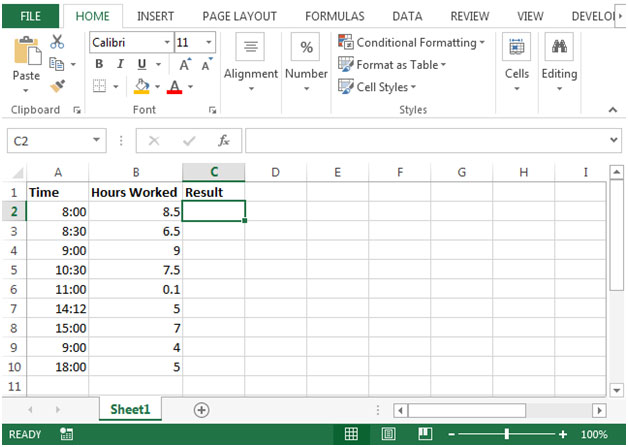• When adding a time value and a number representing hours, we must first divide the number by 24, as shown in the following formula
• In cell C2, the formula would be =A2+B2/24
• We will get the output as follows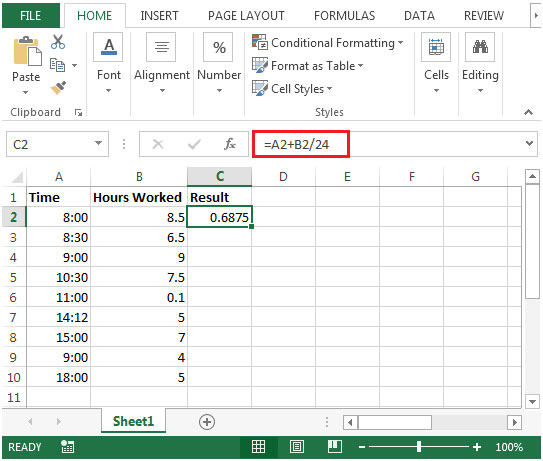• Since the output is not giving us the result in time format.
• We need to use Format cells option.
• Right click on the output cell i.e. cell C2 & select Format cells option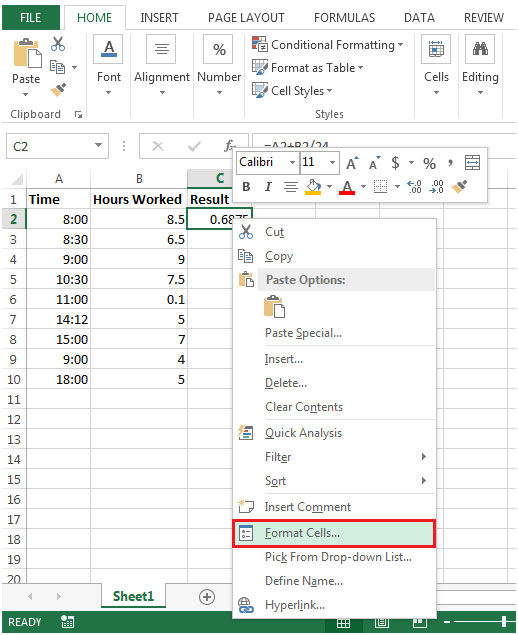• The format cells dialog box will appear.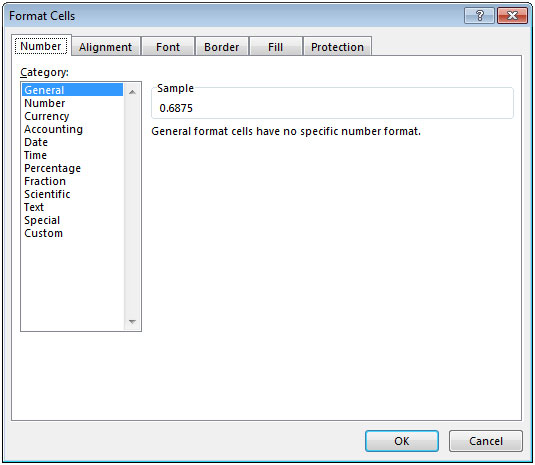• Select Number tab
• In Category box, select Custom.
• In Type box enter [hh]:mm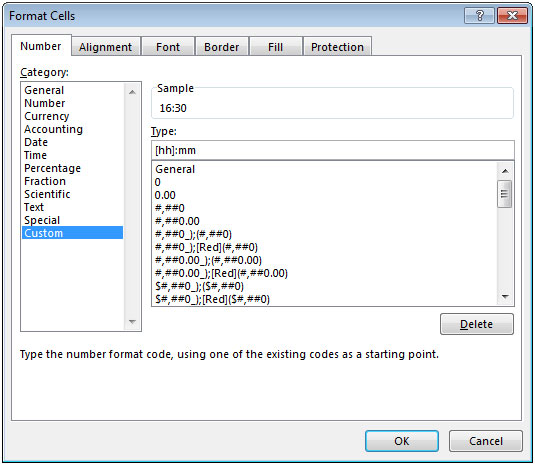• Click on OK. You will see the result in time format.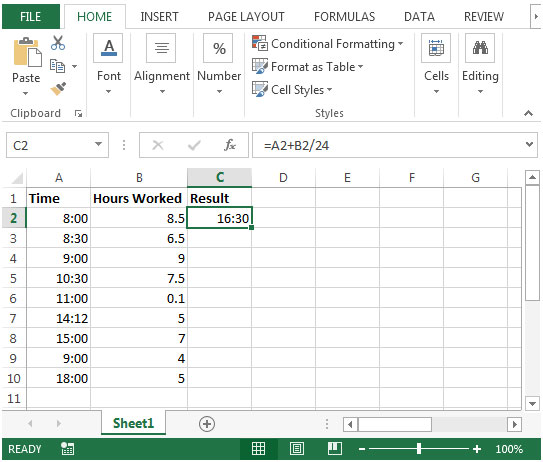• Copying the value in cell C2 to below range C3:C10, we will get the desired output.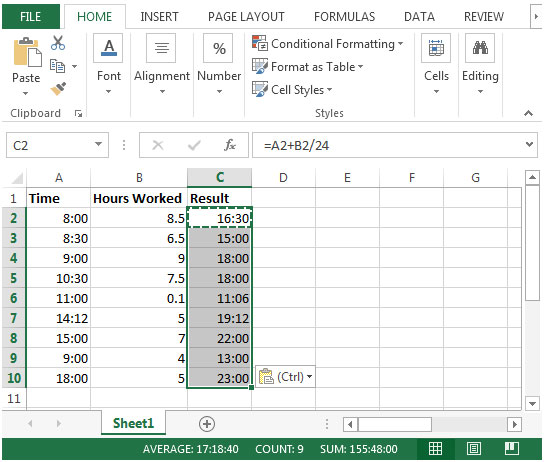1.I have a Macbook microsoft 2010,
and I am trying to add 2:30:00 AM and 853.73 seconds (which has been calculated in the spreadsheet)
I tried converting seconds into hours and adding it this way, and playing around with time format, and such. but I cant seem to be getting a proper answer.
Any help would be appreciated
Thank you

•Hi,